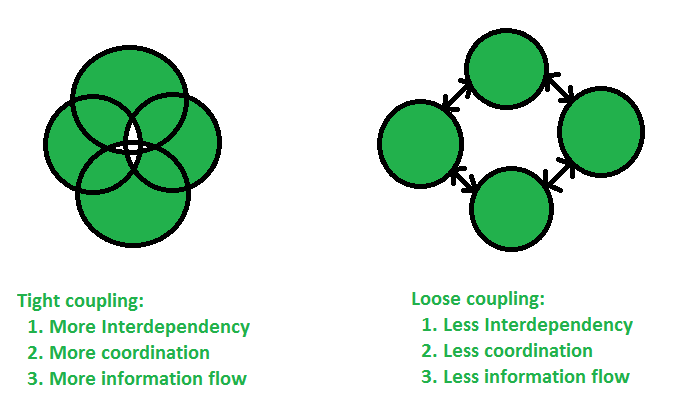# Coupling in Java

• Difficulty Level : Easy
• Last Updated : 05 Feb, 2020

In object oriented design, Coupling refers to the degree of direct knowledge that one element has of another. In other words, how often do changes in class A force related changes in class B.
There are two types of coupling:

1. Tight coupling : In general, Tight coupling means the two classes often change together. In other words, if A knows more than it should about the way in which B was implemented, then A and B are tightly coupled.
Example : If you want to change the skin, you would also have to change the design of your body as well because the two are joined together – they are tightly coupled. The best example of tight coupling is RMI(Remote Method Invocation).

 `// Java program to illustrate ` `// tight coupling concept ` `class` `Subject { ` `    ``Topic t = ``new` `Topic(); ` `    ``public` `void` `startReading() ` `    ``{ ` `        ``t.understand(); ` `    ``} ` `} ` `class` `Topic { ` `    ``public` `void` `understand() ` `    ``{ ` `        ``System.out.println(``"Tight coupling concept"``); ` `    ``} ` `} `

Explanation: In the above program the Subject class is dependents on Topic class. In the above program Subject class is tightly coupled with Topic class it means if any change in the Topic class requires Subject class to change. For example, if Topic class understand() method change to gotit() method then you have to change the startReading() method will call gotit() method instead of calling understand() method.

 `// Java program to illustrate ` `// tight coupling concept ` `class` `Volume  ` `{ ` `     ``public` `static` `void` `main(String args[])  ` `     ``{ ` `         ``Box b = ``new` `Box(``5``,``5``,``5``); ` `         ``System.out.println(b.volume); ` `     ``} ` `} ` `class` `Box  ` `{ ` `     ``public` `int` `volume; ` `     ``Box(``int` `length, ``int` `width, ``int` `height)  ` `     ``{ ` `         ``this``.volume = length * width * height; ` `     ``} ` `} `

Output:

```125
```

Explanation:In the above example, there is a strong inter-dependency between both the classes. If there is any change in Box class then they reflects in the result of Class Volume.

2. Loose coupling : In simple words, loose coupling means they are mostly independent. If the only knowledge that class A has about class B, is what class B has exposed through its interface, then class A and class B are said to be loosely coupled. In order to over come from the problems of tight coupling between objects, spring framework uses dependency injection mechanism with the help of POJO/POJI model and through dependency injection its possible to achieve loose coupling.
Example : If you change your shirt, then you are not forced to change your body – when you can do that, then you have loose coupling. When you can’t do that, then you have tight coupling. The examples of Loose coupling are Interface, JMS.

 `// Java program to illustrate  ` `// loose coupling concept ` `public` `interface` `Topic ` `{ ` `    ``void` `understand(); ` `} ` `class` `Topic1 ``implements` `Topic { ` `public` `void` `understand() ` `    ``{ ` `        ``System.out.println(``"Got it"``); ` `    ``} ` `} ``class` `Topic2 ``implements` `Topic { ` `public` `void` `understand() ` `    ``{ ` `        ``System.out.println(``"understand"``); ` `    ``} ` `} ``public` `class` `Subject { ` `public` `static` `void` `main(String[] args) ` `    ``{ ` `        ``Topic t = ``new` `Topic1(); ` `        ``t.understand(); ` `    ``} ` `} `

Explanation : In the above example, Topic1 and Topic2 objects are loosely coupled. It means Topic is an interface and we can inject any of the implemented classes at run time and we can provide service to the end user.

 `// Java program to illustrate ` `// loose coupling concept ` `class` `Volume  ` `{ ` `     ``public` `static` `void` `main(String args[])  ` `     ``{ ` `         ``Box b = ``new` `Box(``5``,``5``,``5``); ` `         ``System.out.println(b.getVolume()); ` `     ``} ` `} ` `final` `class` `Box  ` `{ ` `     ``private` `int` `volume; ` `     ``Box(``int` `length, ``int` `width, ``int` `height)  ` `     ``{ ` `         ``this``.volume = length * width * height; ` `     ``} ` `     ``public` `int` `getVolume()  ` `     ``{  ` `         ``return` `volume;  ` `          `  `     ``} ` `} `

Output:

```125
```

Explanation : In the above program, there is no dependency between both the classes. If we change anything in the Box classes then we dont have to change anything in Volume class.

Which is better tight coupling or loose coupling?

In general, Tight Coupling is bad in but most of the time, because it reduces flexibility and re-usability of code, it makes changes much more difficult, it impedes test ability etc. loose coupling is a better choice because A loosely coupled will help you when your application need to change or grow. If you design with loosely coupled architecture, only a few parts of the application should be affected when requirements change.
Lets have a look on the pictorial view of tight coupling and loose coupling:Difference between tight coupling and loose coupling

• Tight coupling is not good at the test-ability. But loose coupling improves the test ability.
• Tight coupling does not provide the concept of interface. But loose coupling helps us follow the GOF principle of program to interfaces, not implementations.
• In Tight coupling, it is not easy to swap the codes between two classes. But it’s much easier to swap other pieces of code/modules/objects/components in loose coupling.
• Tight coupling does not have the changing capability. But loose coupling is highly changeable.

This article is contributed by Bishal Kumar Dubey. If you like GeeksforGeeks and would like to contribute, you can also write an article using contribute.geeksforgeeks.org or mail your article to contribute@geeksforgeeks.org. See your article appearing on the GeeksforGeeks main page and help other Geeks.